×

Search anything:

# Unary and Binary Operations in C++

#### Software Engineering C++Operators are the basic building blocks and constitute the essential part of any programming language. With the help of operators we are able to perform multiple operations. Here, in this article we are going to focus on arithmetic operations in C++.

There are various arithmetic operators used for performing the arithmetic operations.

Arithmetic oprerations can be categorized into two types-

• Unary arithmetic operations
• Binary arithmetic operations

# UNARY ARITHMETIC OPERATIONS

Operator that takes a single operand/argument and performs an operation is called unary operator. A unary operation performs an operation with only one operand.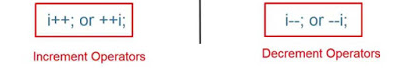• Increment operation : The ‘++’ unary operator is used to increment the value of an integer. When it is placed before variable name, the value of the integer is incremented immediately . It is called pre-increment operator.For example -

CODE:

``````#include<iostream>
using namespace std;

int main()
{
int ans = 0 ,var = 13;

// pre-increment example:
// ans is assigned 11 now since var is updated here itself
cout << "Pre-increament Operation" << endl;
ans = ++ var;
// var and ans have same values = 14
cout << " var is "<< var <<" and ans is "<< ans << endl;

return 0;
}
``````

OUTPUT:

``````Pre-increament Operation
var is 14 and ans is 14
``````

When ‘++’ unary operator is placed after the variable name, its value is not changed/updated for the time being until the execution of the statement containing it. Then the variable value gets updated before the execution of the next statement. It is called the post-increment operator. For example -

CODE:

``````#include<iostream>
using namespace std;

int main()
{
int ans = 0 ,var = 13;

// post-increment example:
// ans is assigned 13 only, var is not updated yet
cout << "Post-increament Operation" << endl;
ans = var ++;
// var becomes 14 now
cout << " var is " << var << " and ans is " << ans << endl;

return 0;
}
``````

OUPUT:

``````Post-increament Operation
var is 14 and ans is 13
``````
• Decrement operation : The '--' unary operator is used to decrement the value of an integer. When it is placed before variable name ,the value of the integer is decremented immediately. It is called pre-decrement operator.For example -

CODE:

``````#include<iostream>
using namespace std;

int main()
{
int ans = 0 ,var = 13;

// pre-decreament example:
// ans is assigned 12 now since var is updated here itself
cout << "Pre-decreament Operation" << endl;
ans = ++ var;
// var and ans have same values = 12
cout << " var is "<< var <<" and ans is "<< ans << endl;

return 0;
}
``````

OUTPUT:

``````Pre-decreament Operation
var is 12 and ans is 12
``````

When ‘--’ unary operator is placed after the variable name, its value is not changed/updated for the time being until the execution of the statement containing it. Then the variable value gets updated before the execution of the next statement. It is called the post-decrement operator. For example -

CODE:

``````#include<iostream>
using namespace std;

int main()
{
int ans = 0 ,var = 13;

// post-decreament example:
// ans is assigned 13 only, var is not updated yet
cout << "Post-decreament Operation" << endl;
ans = var ++;
// var becomes 12 now
cout << " var is " << var << " and ans is " << ans << endl;

return 0;
}
``````

OUPUT:

``````Post-increament Operation
var is 12 and ans is 13
``````

# BINARY ARITHMETIC OPERATIONS

Operator that takes two operands/arguments and performs an operation is called binary operator. A binary operation performs an operation with two operands.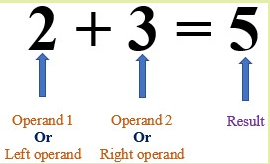There are five types of arithmetic binary operations.

operator used : '+'

2.Multiplication

operator used : '*'

In Multiplication operation, two operands are multiplied.

3.Divison

operator used : '/'

In Divison operation, two operands are divided.

4.Modulus

operator used : '%'

Modulus operation gives the remainder when first operand is divided by the second operand.

5.Subtration

operator used : '-'

In Subtraction operation, two operands are subtracted.

Example:

CODE:

``````#include<iostream>
using namespace std;

int main()
{
int op1 = 15;
int op2 = 3;

int ans = 0;

ans = op1 + op2 ;
cout << "Addition" << " : " << ans << endl;

//Multiplication
ans = op1 * op2 ;
cout << "Multiplication" << " : " << ans << endl;

//Divison
ans = op1 / op2;
cout << "Divison" << " : " << ans << endl;

//Modulus
ans = op1 % op2;
cout << "Modulus" << " : " << ans << endl;

//Subtraction
ans = op1 - op2;
cout << "Subtraction" << " : " << ans << endl;

return 0;
}
``````

OUTPUT:

``````Addition : 18
Multiplication : 45
Divison : 5
Modulus : 0
Subtraction : 12
``````

But there is also one thing i.e to be kept in mind while evaluating the arithmetic operations.
The following table is the Precedence of arithmetic operators.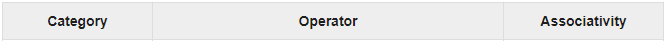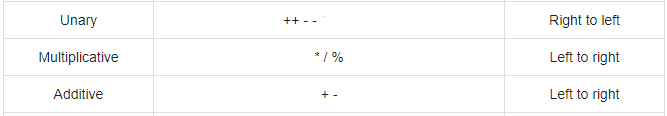The top of the table contains the operators with highest precedence, coming down of the table contains those with lowest at the bottom. Operators having higher precedence will be evaluated first followed by those with lower precedence.

Example : the expression (2 + 3) + 20 + 8 / 4 * 3

(i) 2 is added to 3, yielding result equals 5.
(ii) Then 8 is divided by 4, yielding result equals 2.This result is then multiplied with 3 giving 6 as answer.
(iii) 5 is then added to 20, yeilding result equal to 25.This result is then added to 6, yielding the final result of 31.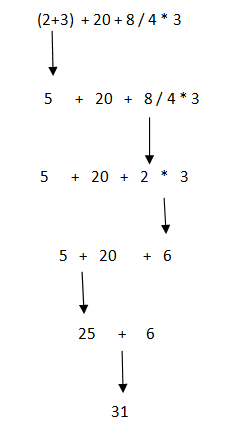## Question

#### What is the value of m? Given m = 605 / 10 + 45 % 7 + 29 % 11;

70
41
2
71
Answer is "70", which will be executed as follows :-
m = (605 / 10) + (45 % 7) + (29 % 11)
m = 60 + 3 +7
m = 70

With this article at OpenGenus, you must have the complete idea of Unary and Binary Operations in C++. Enjoy.